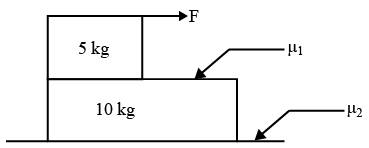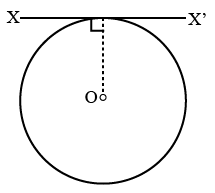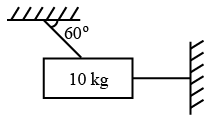# ISRO Scientist or Engineer Mechanical 2016

Instructions

For the following questions answer them individually

Question 11

# The reading ofa spring balance is from 0 to 200 N and is 10 cm long. A body suspended from the spring balance is observed to oscillate vertically at 2 Hz. The mass of the body is nearlyQuestion 12

# A ball is dropped vertically from a height d above the ground. It hits the ground and bounces up to a height d/2. Neglecting the subsequent motion and air resistance, its velocity (v) varies with height (h) above the ground asQuestion 13

# A 10 kW drilling machineis used to drill a bore in a small aluminium block of mass 8 kg. How much is the rise in temperature of the block in 2.5 minutes, assuming 50% of power is used up in heating the block? (specific-heat of aluminum : 0.91 J/($$g^\circ C$$))Question 14

# In the figure shown, the minimum ratio of $$\frac{\mu_1}{\mu_2}$$ so that the masses move together with the application of force F isQuestion 15

# A thin wire of length L and uniform linear mass density $$\rho$$ is bent into a circular loop with centre O as shown in figure. The moment of inertia of the loop about the axis 'XX' isQuestion 16

# A 10 kg mass is hung from 2 light, inextensible strings as shown. The tension in the horizontal string is nearlyQuestion 17

# A block of ice at $$-10^\circ C$$ is slowly heated and converted to steam at $$100^\circ C$$. Which of the following curves represent the phenomena qualitatively?Question 18

# If the temperature of the sun is doubled, the rate of energy received on earth will be increasedby a factor ofQuestion 19

# The transmission of heat by molecular collision is calledQuestion 20

# If two ends of rods of length L and radius r, made of same material are kept at the same temperature difference, which of the following rods conduct most heat per unit time?OR